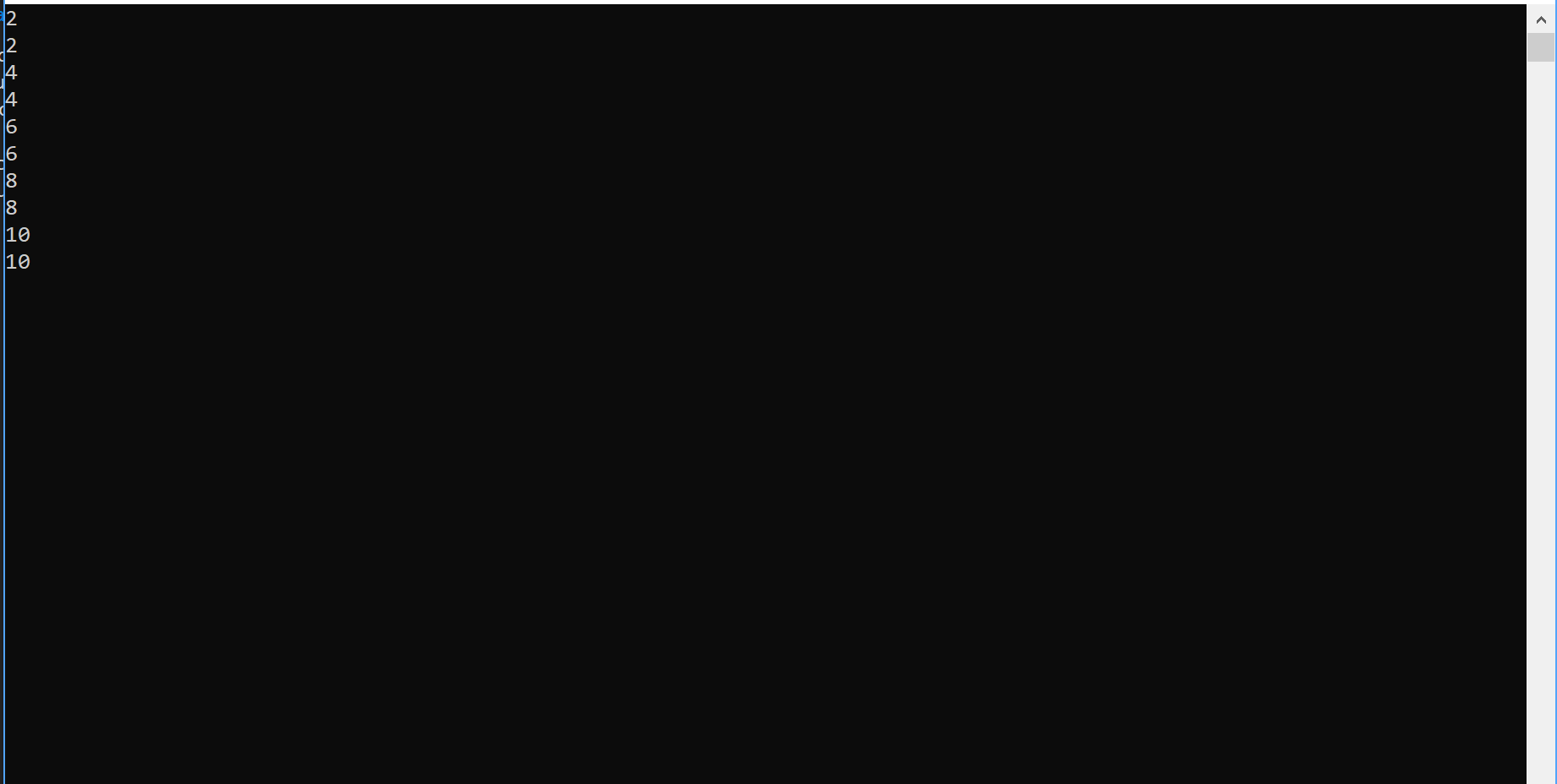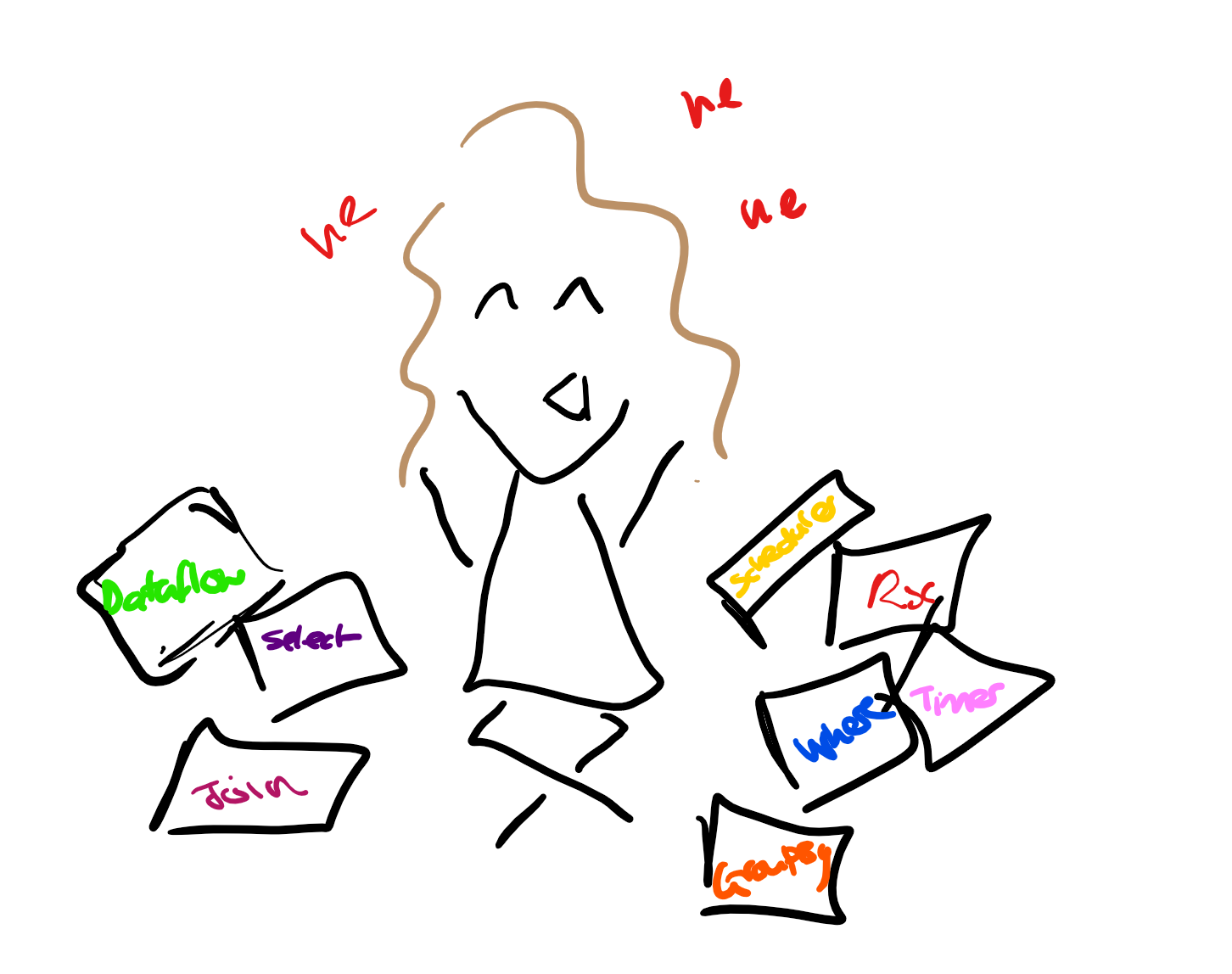In case you missed it… Here's a link to my last blog on understanding Rx (luckily this blog has an internal buffer so if you're just tuning in now, you've not missed your chance)!

## OnNext(Understanding of the Rx operators)

Now one of the most exciting things about Rx is that it has its own implementation of LINQ. This means that you can perform various operations using the same syntax that you would with the standard pull-based collections.

There are a huge amount of operators in Rx to explore, but first, lets get a look under the hood!

## How the operators work…

So, I have one of those brains which makes it rather difficult to just accept something as fact. I really struggled to understand exactly what was happening when you used an operator on an observable sequence, luckily, during my weekly one-on-one with our resident technical fellow (and experienced explainer of complicated things), I got the opportunity to ask just that!

In this blog I'm going to attempt to lay out that explanation, dissecting the `Where` operator as an example.

So… If you were going to write your own where operator, it might look something like the following:

``````static IObservable Where(this IObservable source, Func<TSource, bool> predicate)
{
return new WhereOperator(source, predicate);
}
``````

The static `Where` method is an extension method on an `IObservable`. So that calls to `AnObservable.Where(predicate)` will work as expected. This method then returns an instance of a `WhereOperator` class, which might look something like this (details to follow):

``````private class WhereOperator : IObservable
{

public WhereOperator(IObservable source, Func<T, bool> predicate)
{
this.source = source;
this.predicate = predicate;
}

public IDisposable Subscribe(IObserver observer)
{
...
}

private class Filter : IObserver
{
...
``````

The `WhereOperator` contains an `IObservable`, which is the source to be filtered, and a predicate, which is the condition to filter that source on. So if we wanted just the even numbers from 1 to 10:

``````IObservable<int> sourceNumbers = Observable.Range(1, 10);
IObservable<int> filteredNumbers = sourceNumbers.Where(n => n % 2 == 0);
``````

Then, when you then subscribe to `filteredNumbers` (which is an instance of the `WhereOperator`):

``````public class MyObserver : IObserver<int>
{
public void OnNext(int value)
{
Console.WriteLine(value);
}

public void OnCompleted() { }

public void OnError(Exception error) { }

}

var myObserver = new MyObserver();
filteredNumbers.Subscribe(myObserver);
``````

(This could also be done using an anonymous observer).

A new `Filter`, which implements `IObserver`, is created within the `WhereOperator`'s `Subscribe` method:

``````private class WhereOperator : IObservable
{
public IDisposable Subscribe(IObserver observer)
{
var filter = new Filter(observer, predicate);
return source.Subscribe(filter);
}

...
``````

The internal `Filter` class is instantiated using the observer which wants to subscribe to the filtered source, and the filtering predicate. This filter is then subscribed to the underlying source (in this case, `Observable.Range`). For each value that the range produces, it is passed to the `OnNext` method of the filter.

``````private class Filter : IObserver<T>
{
private IObserver<T> observer;
private Func<T, bool> predicate;

public Filter(IObserver<T> observer, Func<T, bool> predicate)
{
this.observer = observer;
this.predicate = predicate;
}

public void OnNext(T value)
{
if (this.predicate(value))
{
this.observer.OnNext(value);
}
}

public void OnCompleted()
{
this.observer.OnCompleted();
}

public void OnError(Exception error)
{
this.observer.OnError(error);
}
}
``````

When the `OnNext` method of the Filter is invoked, if the predicate is `true` it will call the `OnNext` of the underlying observer. If the predicate is not satisfied then the value will not be passed on.

In this case, the `Filter` created inside the subscribe method has `myObserver` as its observer property, and the predicate passed into the `Where` operator as it's predicate.

So, when each value from `Range` is passed into the filter, it is then passed through the predicate. If the value is even, the predicate returns `true`. If this is the case, the value is passed to `myObserver`'s `OnNext` method and the number is written out to the console. However, if the number is odd then the predicate returns `false` and the value is not passed on to `myObserver`.

In this way only the even numbers are passed to `myObserver`. The original `IObservable` is filtered by the predicate.

A note to remember though, is that all of the numbers in range are still processed, they are just discarded if they do not meet the criteria (in the same way as every number is still checked when you call `Where` on an `IEnumerable`).

So, as all of the work is done each time an observer subscribes to `Range`, this will mean that the numbers 1 to 10 are produced each time someone subscribes.

Now, for something that's just producing the numbers 1 to 10 that's not too much of an issue. But, if the processing was slightly more involved, you may want a way to send the filtered results to multiple observers while only doing the processing once.

## Enter `Publish()`

If you call `Publish` on an observable source, you are returned an `IConnectableObservable`. If you created it yourself it might look a little something like this:

When you subscribe an observer to a connectable observable, that observer is subscribed to the `Subject`. And as we saw in my last blog, it is added to the subject's internal list of observers.

(TL;DR When the subject's `OnNext` method is called, it passes the value in to the `OnNext` of all of the observers in its list).

When you call the `Connect` method, you are telling the connectable observable that everything that needs to subscribe has done so. At this point, it subscribes the subject to the underlying source and items will start being passed to all of the listening observers. In practice you could use it as follows:

``````var myConnectableObservable = new MyConnectableObservable<int>(filteredNumbers);
myConnectableObservable.Subscribe(n => Console.WriteLine(n));
myConnectableObservable.Subscribe(n => Console.WriteLine(n));

myConnectableObservable.Connect();
``````

And equivalently, using `Publish()`:

``````IConnectableObservable<int> multicastFilteredNumbers = filteredNumbers.Publish();

multicastFilteredNumbers.Subscribe(n => Console.WriteLine(n));
multicastFilteredNumbers.Subscribe(n => Console.WriteLine(n));

multicastFilteredNumbers.Connect();
``````

In each of these cases we have subscribed (using anonymous observers) twice to the connectable observable.

The output from either one of these implementations will be as follows (and will only be produced once `Connect` is called):And in each case, the processing to produce the range has only been carried out once.

I had dreams of this blog being an overview of the operators in Rx, however I think we can all agree that I've pushed out enough information for now (not that it's up to you observers)…

Keep an eye out for my next blog where I'll be diving into another of the operators (unless I get distracted again). So, until the next `OnNext` call! (I'm pretty pleased with this analogy)

Oh and here's me... Surrounded by my expansive notes... Laughing at my own puns.### Carmel Eve

#### Software Engineer I